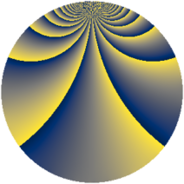# Properties

 Label 1323.2.uLevel $1323$ Weight $2$ Character orbit 1323.u Rep. character $\chi_{1323}(190,\cdot)$ Character field $\Q(\zeta_{7})$ Dimension $444$ Sturm bound $336$

# Related objects

## Defining parameters

 Level: $$N$$ $$=$$ $$1323 = 3^{3} \cdot 7^{2}$$ Weight: $$k$$ $$=$$ $$2$$ Character orbit: $$[\chi]$$ $$=$$ 1323.u (of order $$7$$ and degree $$6$$) Character conductor: $$\operatorname{cond}(\chi)$$ $$=$$ $$49$$ Character field: $$\Q(\zeta_{7})$$ Sturm bound: $$336$$

## Dimensions

The following table gives the dimensions of various subspaces of $$M_{2}(1323, [\chi])$$.

Total New Old
Modular forms 1044 444 600
Cusp forms 972 444 528
Eisenstein series 72 0 72

## Trace form

 $$444q - 72q^{4} + q^{7} + O(q^{10})$$ $$444q - 72q^{4} + q^{7} + 4q^{10} + 2q^{13} - 68q^{16} + 2q^{19} + 2q^{22} - 58q^{25} - 28q^{28} - 12q^{31} - 20q^{34} - 7q^{37} - 50q^{40} - 48q^{43} + 20q^{46} - 23q^{49} + 42q^{52} + 172q^{55} + 76q^{58} - 7q^{61} - 24q^{64} + 18q^{67} - 100q^{70} + 6q^{73} + 268q^{76} - 6q^{79} - 98q^{82} - 60q^{85} + 60q^{88} + 141q^{91} + 152q^{94} + 66q^{97} + O(q^{100})$$

## Decomposition of $$S_{2}^{\mathrm{new}}(1323, [\chi])$$ into newform subspaces

The newforms in this space have not yet been added to the LMFDB.

## Decomposition of $$S_{2}^{\mathrm{old}}(1323, [\chi])$$ into lower level spaces

$$S_{2}^{\mathrm{old}}(1323, [\chi]) \cong$$ $$S_{2}^{\mathrm{new}}(49, [\chi])$$$$^{\oplus 4}$$$$\oplus$$$$S_{2}^{\mathrm{new}}(147, [\chi])$$$$^{\oplus 3}$$$$\oplus$$$$S_{2}^{\mathrm{new}}(441, [\chi])$$$$^{\oplus 2}$$